# Spherical Mirrors

• Last Updated : 12 May, 2021

The light gets reflected from the surfaces. Any surface which is polished or in other words is shiny always acts like a mirror be it of any kind. The observation of light bouncing off or getting back from the surfaces is termed reflection. The light after the case of reflection travels or follows in the same medium from where the ray was incident on the surface. This phenomenon of reflection does not intend to change the velocity of light it only reverses the direction of light incident on it. This can be observed on any surface which is rough or smooth. The path of the reflected ray will depend upon the extent of smoothness of the surface, in the case of smooth surface the reflected ray emerges with the same angle as of incidence and in the latter case suffers irregular reflection and so the reflected ray doesn’t emerge the same as that of incidence angle.

The curved surface we see of a shining spoon can be considered as a curved mirror. The most widely used type of curved mirror is the spherical mirror. The reflecting surface of such mirrors is considered to form a part of the surface of any sphere. That type of mirrors which has their reflecting surfaces spherical, are called spherical mirrors. Let us study that more deeply.

### Spherical Mirrors

A spherical mirror or a mirror that is a part of a sphere is a mirror that has the shape of a piece that is cut out of a spherical surface or material. There exist two types of spherical mirrors which are: concave and convex mirrors.

The History of Spherical Mirrors studied in Human Civilization includes, the first mirrors used by humans were considered most likely pools of water. The earliest known studied and manufactured mirrors were polished stone pieces. Back in 500 AD, Chinese people tended to make spherical mirrors using silver-mercury amalgams. Claudius Ptolemy famous scientist conducted various experiments with curved polished iron mirrors.

### Basic Terms for Spherical Mirrors

There are some common terms that we need to know while studying spherical mirrors, and they are as follows:

• Centre of Curvature: The point in the centre of the mirror surface that passes through the curve of the mirror and has the same tangent and curvature at that point. It is represented by the capital letter C.
• Radius of Curvature: It is considered as the linear distance between the pole and the centre of curvature. It is represented by the capital letter R, R=2f
• Principal axis: An imaginary line that passes through the optical centre and from the centre of curvature of a spherical mirror. All the measurements are based on this line.
• Pole: The midpoint or the centre point of the spherical mirror. It is represented by capital P. All the measurements are made from it only.
• Aperture: An aperture of a mirror is a point from which the reflection of light actually takes place or happens. It also gives an idea about the size of the mirror.
• Principal Focus: Principal Focus can be called the Focal Point also. It is present on the axis of a mirror where the rays of light parallel to the principal axis converge or appear to converge or diverge after reflection.
• Focus: It is any given point on the principal axis where light rays parallel to the principal axis will converge or appear to converge after getting reflected from the mirror.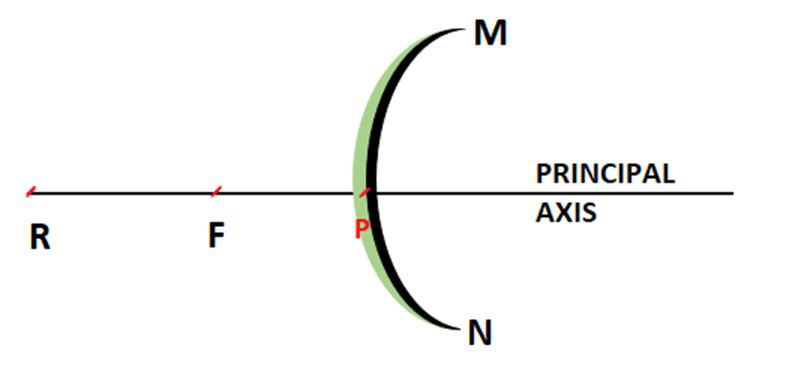### Applications of Spherical Mirrors

They always make upright, enlarged images, and are therefore useful in makeup application or shaving. They are widely used in everyday life as in flashlights and the headlights as they project parallel beams of light, and in the telescopes, as they focus light to produce greatly enlarged images.

A concave mirror is widely used as the reflector in searchlights everywhere, as headlights of motor vehicles, as solar cookers, in torches, and in the table lamps. A convex mirror is greatly used in the automobiles such as in scooters, trucks, and in buses as a rearview mirror to see the traffic behind the vehicle.

### Structure of Spherical Mirrors

A spherical mirror can be a concave or a convex mirror depending upon the surface of reflection. If it is bulged out then it is a convex spherical mirror whereas if it is bent inwards it is termed as a concave spherical mirror.

A typical spherical mirror is a part of a big sphere of which the cut out has been taken. The following diagram describes different parts which are there in a spherical mirror. The definition of these parts is already given above.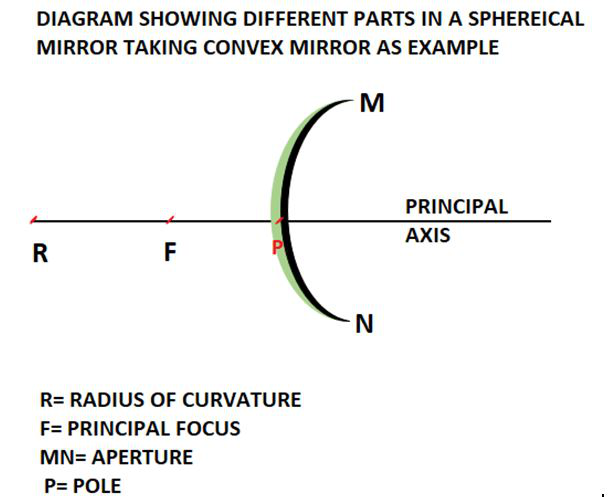### Types of Spherical Mirrors

The reflecting surface or the place where the reflection takes place in case of a spherical mirror may be curved inwards or outwards. As we know based on the type of the reflecting surface there are two very common and widely used spherical mirrors that we use on a daily basis. The following is the diagram showing the concave as well as the convex mirror.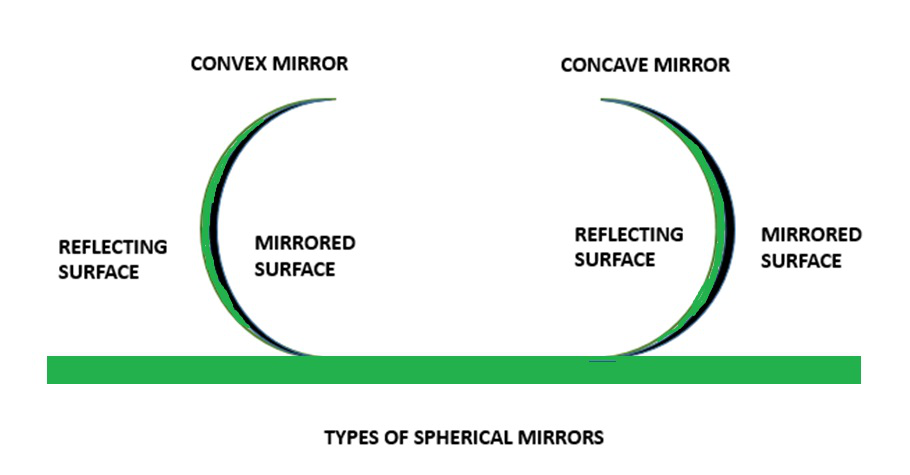### Concave Mirror

A spherical mirror of which the reflecting surface is curved inwards which means that it faces towards the centre of the sphere is known to be a concave mirror. The back of the mirrors is always shaded so that reflection can take place only from the inward bulged surface. The surface of the spoon which is curved inwards can be approximated to a concave mirror. It is also known as the converging mirror as the ray of light after bouncing back from it appears to converge at some points where we can obtain a real, inverted, and enlarged or diminished image based on the location of the object.

Uses of Concave Mirror:

• Converging mirrors are most widely used in shaving because they have reflective and curved surfaces.
• Concave mirror is used in the ophthalmoscope
• These mirrors are also widely used in making astronomical telescopes. In an astronomical telescope, a converging mirror of a diameter of about 5 meters or more is used as the objective.
• Converging mirrors are widely used in headlights of automobiles and in motor vehicles, torchlights, railway engines, etc. as reflectors.
• Large converging mirrors are used to focus the sunlight to produce heat in the solar furnace.

### Convex Mirror

A spherical mirror having its reflecting surface curved outwards is known to be a convex mirror. The back of the mirror is shaded so that reflection only takes place from the outward bulged part. The surface of the spoon which is bulged outwards can be assumed to be a convex mirror. It is also known as a diverging mirror as the light after reflecting through its surface diverges to many directions but appears to meet at some points where the virtual, erect image of diminished size is formed.

Uses of Convex Mirror:

• Convex mirrors used inside buildings so that people can see all around the building at once.
• Convex mirror used in vehicles. Convex mirrors are commonly used as rear-view mirrors in the case of automobiles and vehicles because they can diverge light beams and make virtual images.
• These mirrors are mostly used for constructing magnifying glasses. In industries, to construct a magnifying glass, two convex mirrors are placed back to back.
• Diverging mirrors are also used for security purposes in many places. They are placed near ATMs to let the bank customers check whether someone is behind them or not.
• Convex mirrors are also widely used in various other places for example like as streetlight reflectors because they can spread light over bigger areas.

### Sign Convention for Spherical Mirrors

Usually, if the object is located on the left side of the principal axis from the mirror then the object distance is taken negative. While if it is located on the right side it is taken to be positive. The sign of focal length depends on the type of mirror we are using, as for the concave mirror it is negative and for the convex mirror on the other hand is positive always. It is to be mentioned again that we have to follow the sign conventions strictly to get the correct answer. Heights which are above the principal axis are positive and below are negative.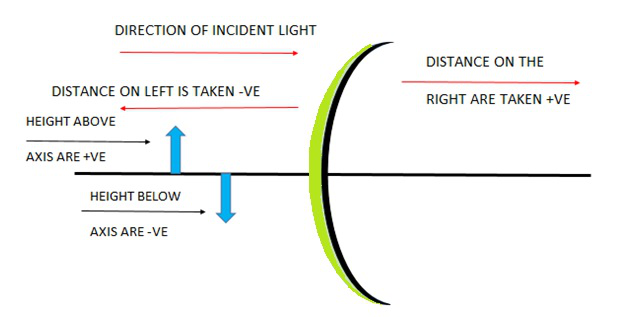## Ray Tracing

The following examples show how rays are traced for various positions of an object from the spherical mirrors:

### When theobject is kept at the infinity in case of concave mirror

As the parallel rays coming from the object converge at the principal focus, F of a concave mirror; after reflection through it. Therefore, when the object is at infinity the image will form at F.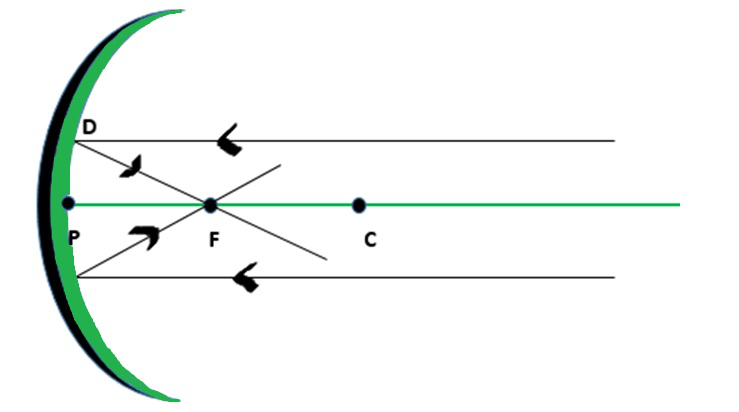Fig: Object at Infinity

Properties of the image formed:

• Point-sized image, highly diminished in size, Real and inverted image.
• The parallel lines which come from the very distant object at infinity after striking the reflecting surface of the concave mirror get reflected back and meet at a point, or we can say in this case converge at a point. This point is known as the principal focus of the concave mirror.

### When the object is kept at infinity in case of convex mirror

Whenever the object is kept at infinity, we observe that a point-sized image is formed at principal focus behind the convex mirror.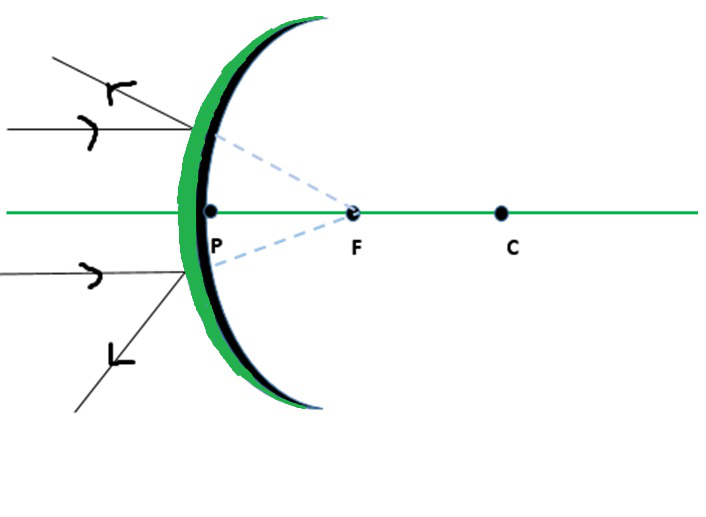Fig: Object at infinity

Properties of image:

• Image formed is highly diminished in size, virtual, and erect.
• The parallel lines which come from the very distant object at infinity after striking the reflecting surface of the convex mirror gets reflected back and appear to meet at a point, or we can say in this case diverge from the surface and appear to meet at a point. This point is known as the principal focus of convex mirror.

### Sample Problem

Question 1: Find out the focal length with sign in case of concave mirror with a radius of curvature 30cm.

Solution:

As we know that R=2f

Where R= radius of curvature of concave mirror, f= focal length of concave mirror

R= 2ff= -15cm (negative indicates that it is a concave mirror.)

Hence, the focal length of the concave mirror is 15cm.

Question 2: Give the types of spherical mirrors.

There are two types of spherical mirrors:

1. Concave mirror which has an inward bent surface.
2. Convex mirror which has a bulged outwards surface.

Question 3: How can you define the principal focus of a concave mirror?

When a parallel beams of light rays are incident on a concave mirror they after reflection through its surface converge at a particular point on the principal axis which is known as principal focus of concave mirror.

Question 4: How can you define the principal focus of a convex mirror?

When a parallel beams of light rays are incident on a convex mirror they after reflection through its surface diverge through its surface but appear to meet at a particular point on the principal axis which is known as principal focus of convex mirror.

Question 5: What is the focal length of the convex mirror if the radius of curvature is 40cm?

Solution:

As we know that R=2f

Where R= radius of curvature of convex mirror, f= focal length of convex mirror

R= 2ff= +20cm (positive indicates that it is a convex mirror.)

Hence, the focal length of the convex mirror is 20cm.

Question 6: What is the radius of curvature of convex mirror if its focal length is 16cm?

Solution:

As we know that R=2f

Where R= radius of curvature of convex mirror, f= focal length of convex mirror

R= 2f

R= 2 x 16cm

R= 32cm

Hence, the Radius of curvature of the convex mirror is 32cm.

My Personal Notes arrow_drop_up